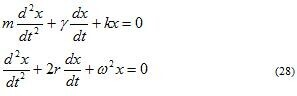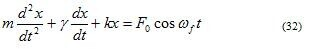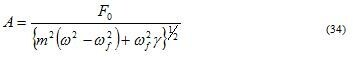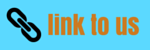# Simple Harmonic Motion

### 9. Damped Oscillations

• Fractional force, acting on a body opposite to the direction of its motion , is called damping force.
• Damping force reduces the velocity and the Kinetic Energy of the moving body.
• Damping or dissipative forces generally arises due to the viscosity or friction in the medium and are non conservative in nature.
• When velocities of body are not high, damping force is found to be proportional to velocity v of the particle i.e.,
Fd=-γv          (27)
where, γ is the damping constant.
• If we take damping into consideration for an oscillator then oscillator experiences
(i) Restoring Force :- F=-kx
(ii) Damping Force :- Fd=-γv
where, x is th edisplacement of oscillating system and v is the velocity of this dispalcement.
• Thus equation of motion of damped harmonic oscillator iswhere,r=(γ/2m) and ω2=k/m
• Solution of above equation is of the form
x=Ae-rtcos(ω't+φ)          (29)
where,
ω'=√(ω2-r2)     (30)
is the angular frequency of the damped oscillator.
• In equation 29 , x is a function of time but it is not a periodic function and because of the damping factor e-rt this function decreases continously with time.

### 10. Driven or Forced Harmonic oscillator

• If an extra periodic force is applied on a damped harmonic oscillator, then the oscillating system is called driven or forced harmonic oscillator, and its oscillations are called forced oscillations.
• Such external periodic force can be represented by
F(t)=F0cosωft          (31)
where, F0 is the amplitude of the periodic force and ωf is th e frequency of external force causing oscillations.
• Differential equation of motion under forced oscillations is• In this case particle will neither oscillate with its free undamped frequency nor with damped angular frequency rather it would be forced to oscillate with angular frequency ωf of applied force.
• When damped oscillator is is set in forced motion, the initial motion is combination of damped oscillation and forced oscillations .
• After certain amount of time the amplitude of damped oscillations die out or becomec so small that they can be ignoredand only forced oscillationd remainand the motion is thus said to reached steady state.
• Solution of equation 32 is
x=Acos(ωft+φ)                (33)
where A is the amplituse of oscollation of forced oscillator and φ is the initial phase.

• In case of forced oscillations both amplitude A and initial phase φ are fixed quantities depending on frequency ωf of applied force.
• Calculations show sthat amplitudeand initial phase
tanφ=-v0/(ωfx0)
where, x0 is displacement of particle at time t=0, the moment driven force is applied and v0 is the velocity of the particle at time t=0.
• When ωf is very close to ω, then m(ω2-(ωf)2 would be much less than ωfγ, for any reasonable value of γ, then equation 34 becomes
A=F0/γωf          (35)
• Thus the maximum possible amplitude for a given driven frequency is governed by the driving frequency and the damping ,and is never infinity.
• This phenomenon of increase in amplitude when the driving force is close to natural frequency of oscillator is called RESONANCE.
• Thus resonance occurs when frequency of applied force becomes equal to natural frequency of the oscillator without damping.